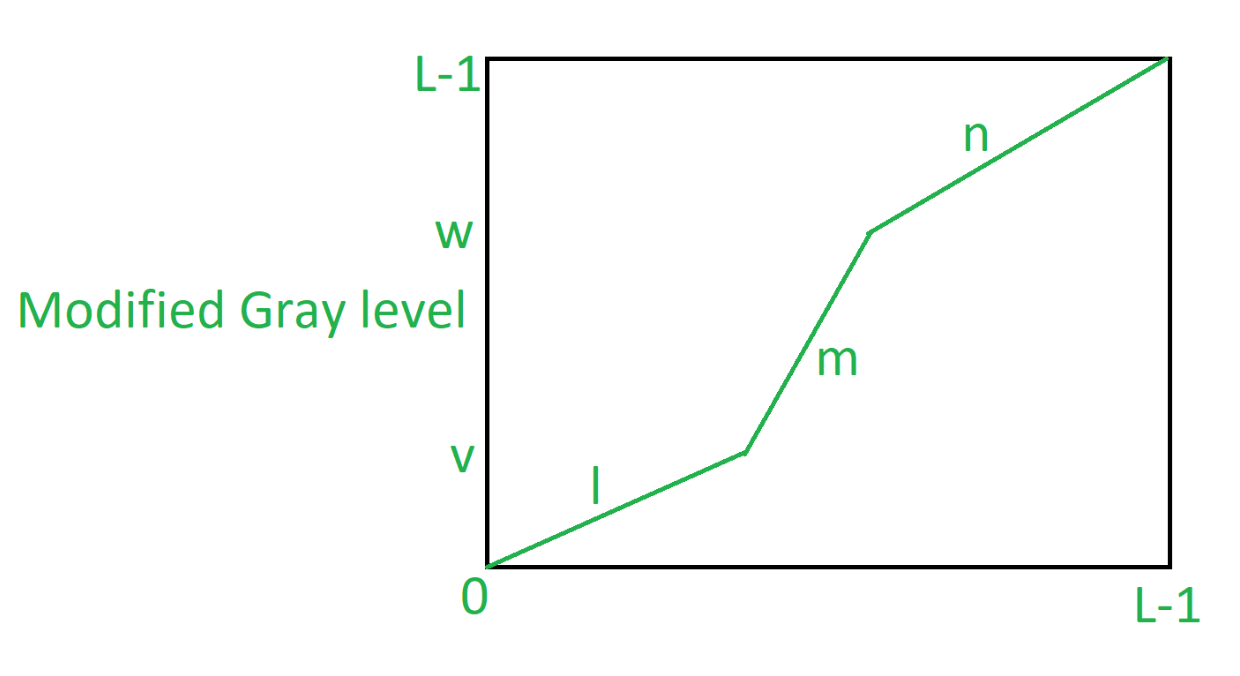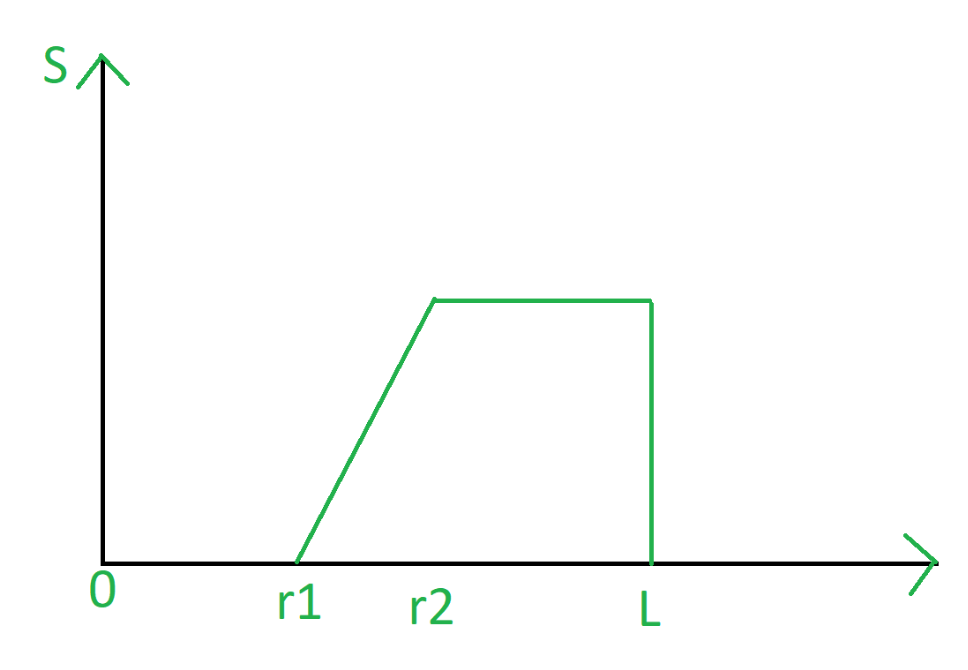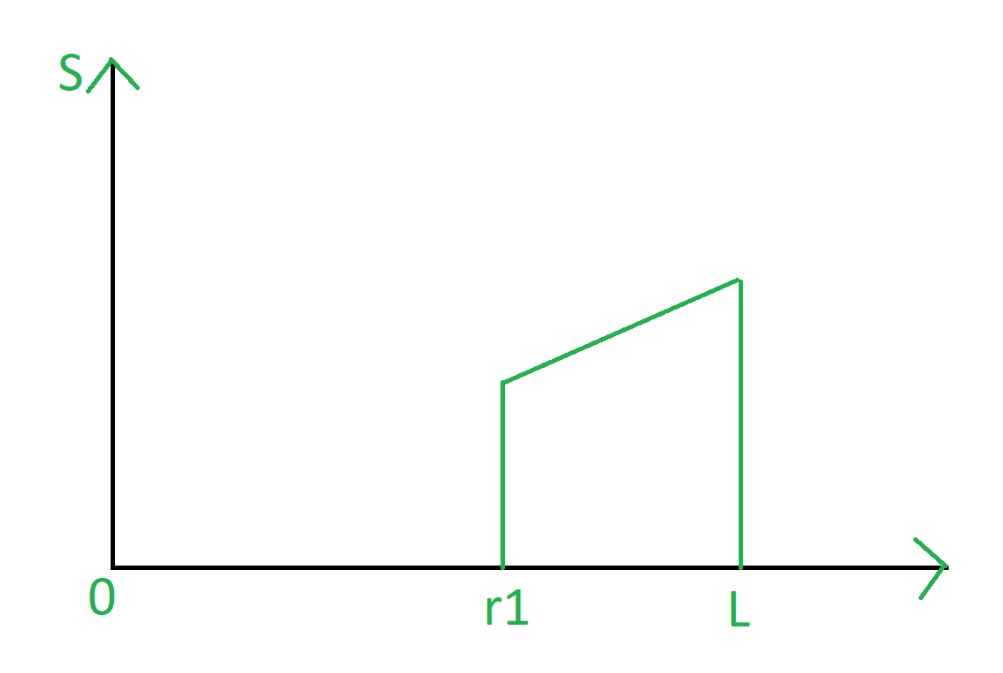Open in App
Not now

# Piece-wise Linear Transformation

• Difficulty Level : Medium
• Last Updated : 05 Dec, 2019

Piece-wise Linear Transformation is type of gray level transformation that is used for image enhancement. It is a spatial domain method. It is used for manipulation of an image so that the result is more suitable than the original for a specific application.

Some commonly used piece-wise linear transformations are:

Contrast Stretching:
Low contrast image occur often due to improper illumination or non-linearly or small dynamic range of an imaging sensor. It increases the dynamic range of grey levels in the image.

Contrast Stretching Transform is given by:

```S = l.r, 0 <= r < a
S = m.(r-a) + v, a <= r < b
S = n.(r-b) + w, b <= r < L-1
where l, m, n are slopes ```Clipping:
A special case of contrast stretching is clipping where l=n=0. It is used for noise reduction when the input signal is known. It puts all grey levels below r1 to black(0) and above r2 to white(1).Thresholding:
Another special case of contrast stretching is thresholding where l=m=t. It is also used for noise reduction. It preserves the grey levels beyond r1.Grey level slicing:
Highlighting a specific range of grey level in an image.

Case-I:

• To display a high value for all grey levels in the range of interest.
• To display a low value for all grey levels.

Case-II:

• Brighten the desired range og grey level.
• Preserve the background quality in the range.

Bit Extraction:
An 8-bit image can be represented in the form of bit plane. Each plane represents one bit of all pixel values. Bit plane 7 contains the most significant bit (MSB) and bit plane 0 contains least significant bit (LSB). The 4 MSB planes contains most of visually significant data. This technique is useful for image compression and steganography.

My Personal Notes arrow_drop_up